## Part 3, Trapezoid Waveform Notes

J. Donald Tillman
1 March 2004Since the trapezoid waveform does not have a real history in electronic music, I thought it would be a good idea to define it a little more completely and investigate some of its properties.

## Trapezoid waveform specifics

The trapezoid wave ramps linearly to a positive reference point, holds at the reference point, ramps linearly to a negative reference point, holds at the negative reference point, and repeats.  A trapezoid wave is like a cross between a triangle wave and a square wave; effectively a slewing square wave.  The waveform is symmetrical, meaning that the time spent slewing positive, holding positive, slewing negative, and holding negative are all equal, and that the slope of the positive ramp is equal and opposite the slope of the negative ramp.

The waveform for this article is normalized so that it has a value that ranges from -1.0 to +1.0, a frequency of 1.0 rad/sec, and a phase such that its value crosses 0.0 in the positive direction at x = 0.0.

Here is a drawing: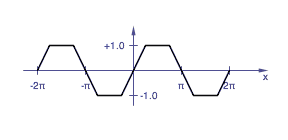Normalized trapezoid waveform.

## Harmonic content

Electronic music folks are invariably interested in the harmonic content of waveforms.  If the waveform is used as a sound source, our sense of hearing interprets the harmonic content far better than the graphic shape of the waveform.

The Fourier components of a trapezoid waveform are:(I've included a full derivation at the end of this article for the folks who appreciate that sort of thing.  You know who you are.)

The first observation is that the waveform contains only odd harmonics.  You can tell that by inspection if you know which symmetries to look for.  The situation is very similar to the square wave and triangle wave in that regard.

The second observation is that the harmonic content looks very familiar if you know the harmonic content of a triangle wave.  Here is the equivalent triangle wave: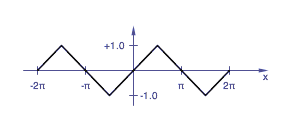Normalized triangle waveform.

The Fourier components of this triangle wave are:(The derivation for this can also be found at the end of this article.)

Both the trapezoid wave and the triangle wave have the same harmonic structure; they both contain only odd harmonics and the amplitude of each harmonic is inversely proportional to the square of the harmonic number.

The polarities of the harmonics are different — the triangle wave alternates polarities on each odd harmonic while the trapezoid wave alternates polarities on sucessive pairs of odd harmonics.  For the triangle wave, the alternating polarities of the odd harmonics mean that the harmonic peaks reinforce each other at the 90 degree and 270 degree points of the waveform.  For the trapazoid wave, the alternating polarities of the pairs of odd harmonics flatten the peaks of the wavesform.

Since our hearing is not especially sensitive to phase, the trapezoid and triangle waveforms sound pretty much alike.  That is not to say you can substitute one for the other in all cases.  For instance, running a triangle wave through a circuit with gradual clipping, such as a Moog ladder filter or a diff amp based VCA, will warp the shape of the triangle wave toward a sine wave with less harmonic content, while the same situation will warp the shape of a trapazoid wave toward a square wave with more harmonic content.

## Peak to RMS ratio

The constants in the trapezoid and triangle equations also differ.  Both equations are for peak voltage swings of -1.0 V to +1.0 V, but with the harmonic polarities of the trapezoid wave combining towards a flatter waveform, the levels of the harmonic components of the trapezoid can be proportionally greater.

The ratio of the peak voltage (0 to peak) to rms voltage of the trapezoid waveform can be calculated and compared with other standard electronic music waveforms:

Waveform Vpeak/Vrms Vrms/Vpeak

triangle 1.732 0.577
sin 1.414 0.707
trapezoid 1.224 0.817
square 1.000 1.000

## Triangle from Trapezoid

Another interesting and useful relationship between trapezoid and triangle waveforms is that it is possible to create a triangle wave by algebraically averaging two trapezoid waves 90 degrees apart.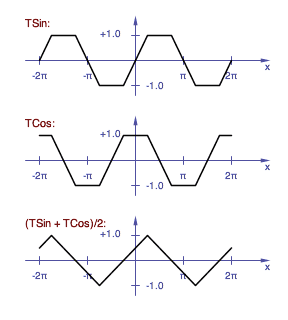Averaging a Trapezoid Sin and a Trapezoid Cosine creates a Triangle wave.

Given the very similar spectrums of the two waveforms, I think it's interesting that adding two phases of a trapezoid wave would have the effect of inverting the polarity of some of the harmonics — we might expect some of the harmonics to add and some to subtract, but not for some of the harmonics to flip polarity.  Because the two trapezoid waveforms are 90 degrees apart, and the phase of the resulting triangle waveform is halfway between the two trapezoids, the odd harmonics branch away in positive and negative directions from the phase of the fundamental at odd multiples of 45 degrees.  At ±45 degrees they're positive, at ±135 degrees they're negative, at ±225 degrees they're still negative, at ±315 degrees they're positive again, and so forth.

## Trapezoid from Triangle

Likewise you can create a trapezoid wave by adding two triangle waves 90 degrees apart: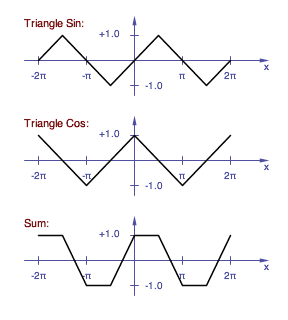Adding two triangle waves to create a trapezoid wave.

## Derivation of Triangle Wave Harmonics

I'm placing the Fourier analysis material in the back so it won't scare people away.

The Fourier series represents a periodic waveform of a given frequency as a sum of sine and cosine functions that are multiples of the fundamental frequency: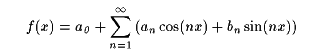Where
f(x) is the function in question
a0 is the dc component
an is the level of each cosine wave
bn is the level of each sine wave
x is the independant variable,
or the time in seconds for a 1 radian/second waveform,
or 2 π f t for a waveform of arbitrary frequency

The formulas for determining the values of the an and bn coefficents are called the Euler Fomulas.  They are: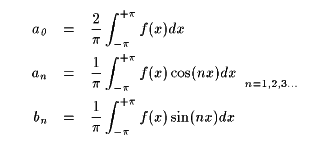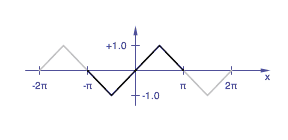Normalized triangle waveform for Fourier analysis.

We can define a 1.0 Hz triangle wave with a -1.0 V to +1.0 V peak-to-peak voltage swing as:The process is a lot easier if we take advantage of the symmetry of sine and cosine waves on the left and right sides of the y axis.  A function g(x) is said to be odd if g(-x) = -g(x).  And for odd functions, the left and right side contributions to the cosine coefficients exactly cancel leaveing the values of an at zero.  Likewise, the contributions of the left and right sides to the sine coefficients are exactly eaual, so you can save some work by calculating one side and doubling it.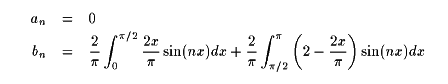To proceed form here you'll need to know the intregral of x sin(x).  You can look this up in a suitably complete table of integrals, derive it by "integration by parts", or you can trial-and-treak derivitives and get it pretty quickly.Solving the integrals: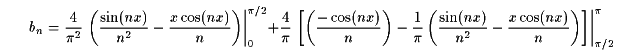Then plug in the values at the endpoints: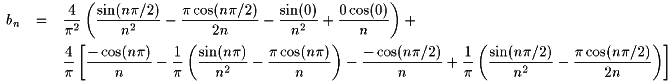Referring to the terms as 1 through 10 above:

terms 1 and 9 add together
terms 2 and 10 add up to cancel term 8
terms 3 and 4 are zero
term 5 and 7 cancel
term 6 is zero because the sin() function is zero for all multiples of π

So this reduces to:The sin(nπ/2) factor repeats a series 1, 0, -1, 0, in a loop, so bn could be represented as: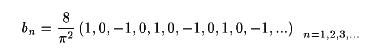And the triangle waveform represented as a Fourier series is:## Derivation of Trapezoid Harmonics

The trapezoid harmonics are determined in a manner very similar to the triangle harmonics.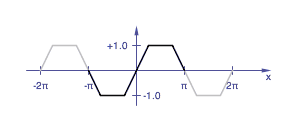Normalized trapezoid waveform for Fourier analysis.

We can define a 1.0 Hz trapezoid wave with a -1.0 V to +1.0 V peak-to-peak voltage swing as:As with the triangle, we can take advantage of the symmetry of the situation.  The trapezoid is also an odd function, and so the cosine coefficients (an, n = 0, 1, 2...) are zero, and the sine coefficients can be determined as twice the value of integrating half the range, 0 through π.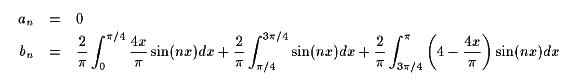Solving the integrals: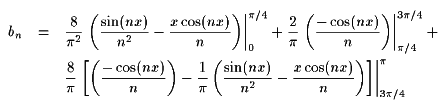Plugging in the values at the endpoints: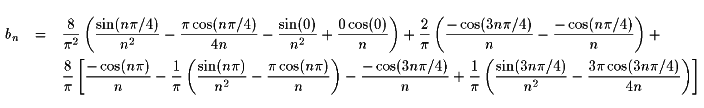Referring to the terms as terms 1 through 12 above:

term 1 stays
term 2 and term 6 cancel
term 3 is zero and term 4 is zero
term 5 and term 12 add together to cancel term 10
term 7 and term 9 cancel
term 8 is always zero
term 11 stays

So this equation reduces, thankfully, to:The sin(n π/4) and sin(3n π/4) terms together create this pattern: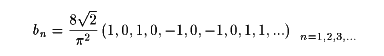So the trapezoid waveform presented as a Fourier series is:Copyright 2003, J. Donald Tillman
don@till.com
http://www.till.com